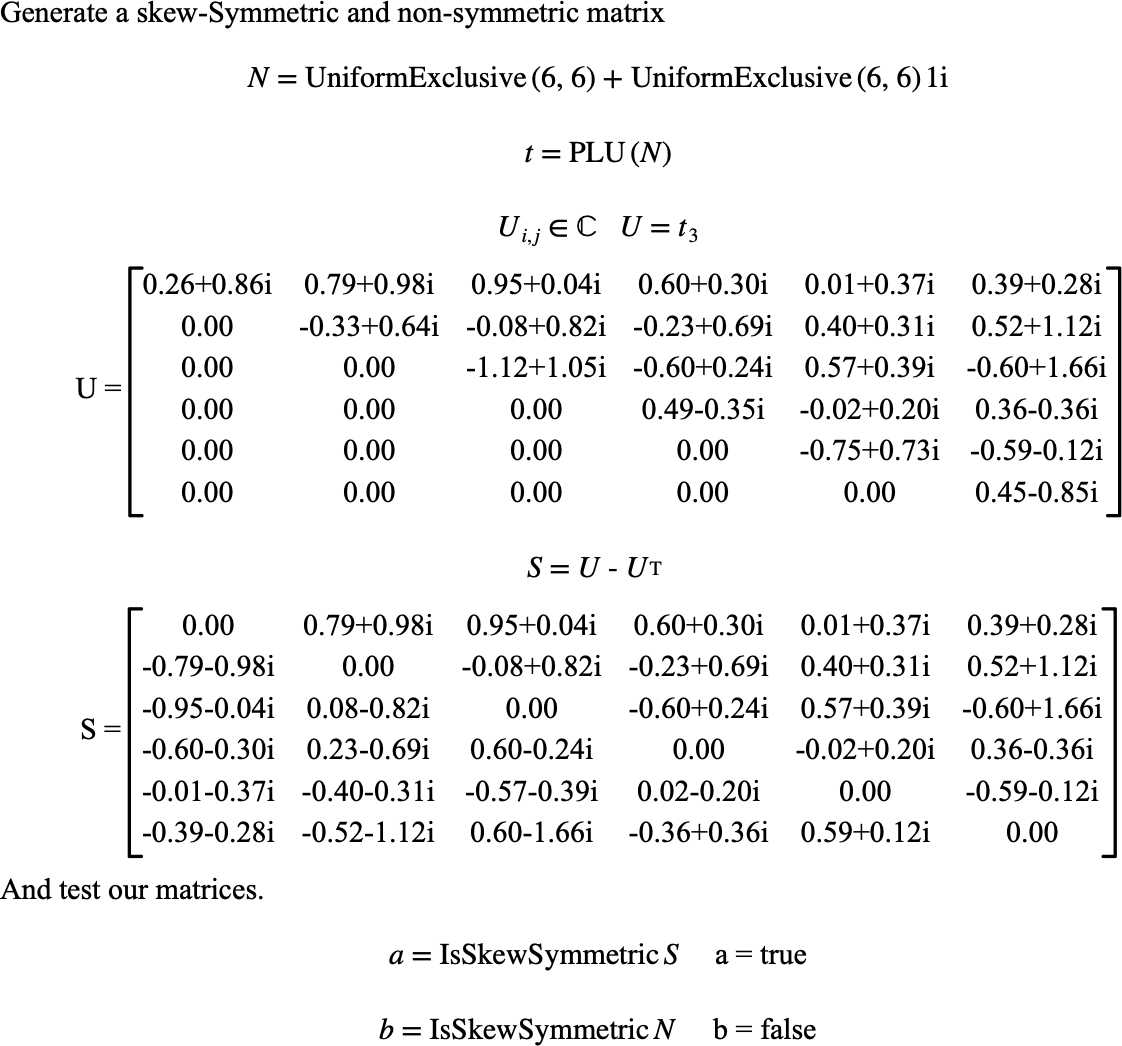# $$\text{IsSkewSymmetric}$$¶

You can use the $$\text{IsSkewSymmetric}$$ function to determine if a matrix is skew symmetric.

You can use the \isssymmetric backslash command to insert this function.

The following variants of this function are available:

• $$\text{boolean } \text{IsSkewSymmetric} \left ( \text{<matrix>} \right )$$

• $$\text{boolean } \text{IsSkewSymmetric} \left ( \text{<matrix>}, \text{<relative tolerance>} \right )$$

The $$\text{<matrix>}$$ parameter is the matrix to be tested. The optional $$\text{<relative tolerance>}$$ parameter specifies the tolerance to apply when comparing values. If not specified, the $$\text{IsSkewSymmetric}$$ function will use a tolerance of $$1.0 \times 10^{-8}$$.

A matrix, $$M$$, is considered skew symmetric if the matrix is square and $$M _ { i, j } = - M _ { j, i }$$. This function relaxes this constraint by allowing a test within a given tolerance. The $$\text{IsSymmetric}$$ function considers a matrix to be skew symmetric if the matrix is square and:

$\left \vert M _ { i,j } + M _ { j,i } \right \vert \leq \frac{1}{2} \left \vert M _ { i,j } - M _ { j,i } \right \vert t _ {rel}$

For all $$1 \leq i \leq \text{nrows} \left ( M \right )$$ and $$1 \leq j \leq i$$ where $$t _ {rel}$$ is the relative tolerance. Note that this test is only applied if $$M _ { i,j } \ne - M _ { j,i }$$.

Figure 161 shows the basic use of the $$\text{IsSkewSymmetric}$$ function.Figure 161 Example Use Of The IsSkewSymmetric Function